Scientific and technical journal

### 2009### Contacts

F. Sсorina Gomel State University
Sovetskaya Str., 104, 246019, Gomel,
Republic of Belarus

Phones:
+375 (232) 51-00-77
+375 (232) 51-03-21
+375 (29) 678-62-50

E-mail:
pfmt*gsu.by

Website:
http://pfmt.gsu.by

## MATERIALS: № 2 (31) 2017

PHYSICS

#### Akhramenko N.A. Gravitational defect of massive ball

The resulting expression defining the gravitational mass defect of massive ball is given. It is shown that the gravitational mass defect in-creases with increasing density and the mass of the ball. The resulting ratio is a generalization for magnitudes of the gravitational mass defect in the non-relativistic case.

Keywords: theory of gravitation, massive ball, gravitational mass defect.

N.A. Akhramenko – Belarusian State University of Transport, Gomel

#### Girgel S.S. Generalized asymmetric of Bessel – Gaussian beams of the continuous order

The new solutions of the parabolic equation featuring the generalized asymmetric of Bessel – Gaussian beams of the continuous order are offered. They are characterized by five free continuous parametres and possess a spiral wavefront. Restrictions on these parametres at which explored fractional beams transfer terminating power are discovered. Pictorial modeling of such beams which confirms the main analytical calculations is fulfilled.

Keywords: asymmetric beams, beams of Bessel – Gaussian, a square intergrability.

S.S. Girgel – Department of Physics, F. Scorina Gomel State University

#### Grishechkin Yu.A., Kapshai V.N., Danilchenko M.S. Relativistic bound s-states problem for superposition of two potentials «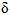-sphere» type

The exact solutions of relativistic two-particle quasipotential type equations for bound states in the case of the potential «-sphere» in the relativistic configuration representation and in the case of the superposition of two such potentials are considered. The signs of the coefficients in the-potentials that allow the existence of bound states and the maximum possible number of this states are defined on the basis of the analysis of the energy quantization conditions.

Keywords: relativistic two-particle equation, relativistic configurational representation, delta-function potential, bound state, energy quantization condition.

Yu.A. Grishechkin – Department of Physics, F. Scorina Gomel State University

V.N. Kapshai – Department of Physics, F. Scorina Gomel State University

M.S. Danilchenko – Department of Physics, F. Scorina Gomel State University

#### Kalosha L.A., Gaida L.S., Komar V.N., Zaerko D.V. Modeling of dielectric gratings to control the redistribution of energy in the spectrum of the scattered microwave optical and electromagnetic fields

The choice of the periodic structures based on hollow dielectric rods with filling, which offer the possibility to vary the dielectric constant is considered. The simulation results obtained for scattering of microwave and optical ranges electromagnetic radiation in equidistant structures based on dielectric rods with variable electrophysical parameters are given. The effect of variations in the dielectric constant of the structural components on the energy redistribution of harmonics of the scattered electromagnetic radiation is demonstrated.

Keywords: periodic dielectric structures, dielectric constant, s-parameter changes, control of the propagation characteristics of microwave and optical ranges electromagnetic radiation.

L.A. Kalosha – Grodno State College of Engineering, Technology and Design

L.S. Gaida – Y. Kupala Grodno State University

V.N. Komar – Y. Kupala Grodno State University

D.V. Zaerko – Y. Kupala Grodno State University

#### Rudenkov A.S., Rogachev A.V., Piliptsov D.G., Xiaohong Jiang, Fedosenko N.N. Influence of carbide-forming metals nature on the phase composition and structure of alloyed carbon coatings

Structural-phase features of carbon coatings deposited from impulse cathode plasma doped with titanium and chromium are determined. It is established that metal-containing formations with an average size of 40..90 nm are formed in the volume of an amorphous carbon matrix during electric arc evaporation of metals. The introduction of chromium into the carbon matrix leads to an increase in the content of carbon atoms in the sp3 state, doping the same carbon coating with titanium helps reduce the number of atoms with sp3 hybridization, increase in the size and ordering of Csp2 clusters.

Keywords: carbon coatings, alloyed, titanium, chromium, phase composition, morphology.

A.S. Rudenkov – Department of Physics, F. Scorina Gomel State University

A.V. Rogachev – Department of Physics, F. Scorina Gomel State University

D.G. Piliptsov – Department of Physics, F. Scorina Gomel State University

Xiaohong Jiang – Nanjing University of Science and Technology

N.N. Fedosenko – Department of Physics, F. Scorina Gomel State University

MATHEMATICS

#### Belokon L.M. Intersections of maximal subgroups in a finite group in connection with the local formations and a generally abnormally full subgroup m-functor

Let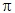be a set of primes. The sufficient conditions that must satisfy a local formation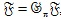, a finite group G and a subgroup m-functor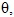under whichalso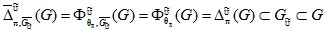ifis radical, are achieved. As the consequences of the main results there were obtained the assertions for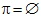and corresponding local formations.

Keywords: maximal subgroups of finite groups, local and local radical formations, subgroup m-functor.

L.M. Belokon – Mogilev State University of Food Technologies

#### Bin Hu, Jianhong Huang, Skiba A.N. Finite groups whose n-maximal subgroups are generalized S-quasinormal

Let G be a finite group and M a subgroup of G. Then M is called: modular in G if the following conditions are held: (i)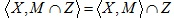for all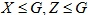such that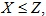and (ii)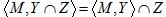for all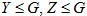such that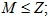quasinormal (respectively S-quasinormal) in G if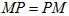for all subgroups (respectively for all Sylow subgroups) P of G. We say that M is a generalized subnormal (respectively generalized S-quasinormal) subgroup of G if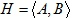for some modular subgroup A and subnormal (respectively S-quasinormal) subgroup B of G. If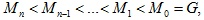where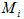is a maximal subgroup of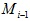for all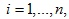then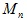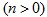is an n-maximal subgroup of G. In this paper, we study finite groups whose n-maximal subgroups are generalized subnormal or generalized S-quasinormal.

Keywords: finite group, S-quasinormal subgroup, modular subgroup, generalized subnormal subgroup, generalized S-quasinormal subgroup.

Bin Hu – Jiangsu Normal University, Xuzhou

Jianhong Huang – Jiangsu Normal University, Xuzhou

A.N. Skiba – Department of Mathematics, F. Scorina Gomel State University

#### Dergacheva I.M., Shabalina I.P., Zadorozhnyuk E.A. A criterion for a finite group to belong a saturated formation

We prove the following result: Let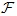be a hereditary saturated formation of p-soluble groups containing all p-supersoluble groups such that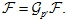Let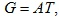where A is a Hall-subgroup of G,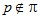and T is a p-supersoluble subgroup of G. Suppose that for a Sylow p-subgroup P of T we have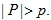If A permutes with a Hall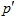-subgroup of T and with all maximal subgroups V of P such that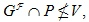then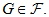Keywords: finite group, saturated formation, p-soluble group, p-supersoluble group, Hall subgroup.

I.M. Dergacheva – Belarusian State University of Transport, Gomel

I.P. Shabalina – Belarusian State University of Transport, Gomel

E.A. Zadorozhnyuk – Belarusian State University of Transport, Gomel

#### Zhogal S.P., Zhogal S.I., Klimenko A.V. On existence and uniqueness of solutions of one complex stochastic differential system with delay

Problems of the existence and uniqueness of solutions of systems of differential equations containing a stochastic differential equation in partial derivatives of hyperbolic type and ordinary stochastic differential equations with delay connected with each other by retarded connections are investigated.

Keywords: stochastic differential equations, delay, stochastic partial differential equations, units with lumped parameters, units with distributed parameters.

S.P. Zhogal – F. Scorina Gomel State University

S.I. Zhogal – Belarusian State University of Transport, Gomel

A.V. Klimenko – F. Scorina Gomel State University

#### Kovaleva V.A. Finite groups with given generalized maximal subgroups (Review). II. From the maximal chains to the maximal pairs

Let G be a finite group. Recall that a maximal chain of G of length n is a chain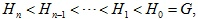such that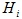is a maximal subgroup of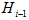for every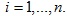In this review we continue the analysis of the most famous papers in which finite groups with given maximal chains are developed.

Keywords: finite group, maximal chain, n-maximal subgroup, maximal pair of subgroups.

V.A. Kovaleva – Department of Mathematics, F. Scorina Gomel State University

#### Semenchuk V.N., Selkin M.V., Selkin V.M. On finite groups with generalized subnormal subgroups

The structure of finite groups with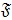-subnormal subgroup is studed.

Keywords: finite group,-subnormal subgroup, saturated formation, hereditury formation.

V.N. Semenchuk – Department of Mathematics, F. Scorina Gomel State University

M.V. Selkin – Department of Mathematics, F. Scorina Gomel State University

V.M. Selkin – Department of Mathematics, F. Scorina Gomel State University

#### Sidortsov M.V., Drapeza A.A., Starovoitov A.P. Asymptotics of Hermite – Pade degenerate hypergeometric functions

The asymptotic behavior of diagonal Hermite – Pade polynomials and diagonal Hermite – Pade approximations of type II for the system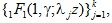consisting of degenerate hypergeometric functions in which while the rest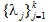are the roots of the equation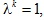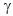– is a complex number belonging to the set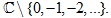was stated. The theorems complement known results of H. Pade, D. Braess, A.I. Aptekarev, H. Stahl, F. Wielonsky, W. Van Assche, A. B. J. Kuijlaars, A.P. Starovoitov, obtained for the case, where the– different real numbers.

Keywords: Hermite integrals, Hermite – Pade polynomials, Hermite – Pade approximations, asymptotic equality, degenerate hyper-geometric functions.

M.V. Sidortsov – Department of Mathematics, F. Sсorina Gomel State University

N.A. Starovoitova – F. Sсorina Gomel State University

A.P. Starovoitov – Department of Mathematics, F. Sсorina Gomel State University

#### Yakubovich O.V., Letunovich Y.E., Evdokimovich V.E. Open queueing network with quarantine node

An open Markov queueing network with quarantine node is considered. Customers arrive in usual exponential nodes and are verified for standard. If a customer does not comply with the standard it is sent to the quarantine node, where its quality is restored. After that the customer continues to move through the network. The conditions of ergodicity and an analytical view of stationary distribution of the network states probabilities are found.

Keywords: queueing network, quarantine node, ergodicity, stationary distribution.

O.V. Yakubovich – Department of Mathematics, F. Sсorina Gomel State University

Y.E. Letunovich – Department of Mathematics, F. Sсorina Gomel State University

V.E. Evdokimovich – Belarusian State University of Transport, Gomel

#### Yarovaya A.V. Forced vibrations of circular sandwich plates under local suddenly applied loads

Axisymmetric transverse vibrations of a circular elastic three-layer plate under the influence of local surface, force and torque loads are considered. The hypothesis of a broken normal is accepted for the kinematics description of asymmetrical on the thickness of package. Kirchhoff's hypotheses are valid in the carrier layers. In a comparatively thick lightweight aggregate, the normal remains straight, but rotates by some additional angle. Analytical solutions are obtained by expanding the series in terms of a system of proper orthonormal functions. Their numerical analysis is made.

Keywords: three-layer circular plate, axisymmetric vibrations, local suddenly applied loads.

A.V. Yarovaya – Belarusian State University of Transport, Gomel

INFORMATION SCIENCE

#### Listopad N.I., Vorotnitsky Y.I., Bortnovsky V.V., Hayder A.A. Multi-criterial routing of information flows

Traditionally, path selection within routing is formulated as the shortest path optimization problem. In this paper, multi-criteria routing based on a mixed weight is considered. It is shown how this approach can be used to develop two heuristic algorithms for searching the optimal path with minimum delay, minimum delay variation, providing the given bandwidth, minimum loss probability and minimum cost of information transmission.

Keywords: multi-criteria routing, mixed weight, delay, delay variation, loss probability, bandwidth, shortest path, Dijkstra’s Algorithm, cost of information transmission.

Y.I. Vorotnitsky – Belarusian State University, Minsk

V.V. Bortnovsky – Belarusian State University of Informatics and Radioelectronics, Minsk

A.A. Hayder – Belarusian State University of Informatics and Radioelectronics, Minsk